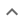•# Power equations

We think you wrote:

###This solution deals with power equations.

Solution found9a^2

## Step by Step Solution## Step  1  :

#### Equation at the end of step  1  :


Step  2  :

#### Equation at the end of step  2  :




## Step  3  :

            (2•32a3b2)
Simplify   ——————————
2ab2


#### Dividing exponential expressions :

a3 divided by a1 = a(3 - 1) = a2

#### Canceling Out :

Canceling out b2 as it appears on both sides of the fraction line

#### Canceling Out :

Canceling out  2  as it appears on both sides of the fraction line

## Final result :

  9a2